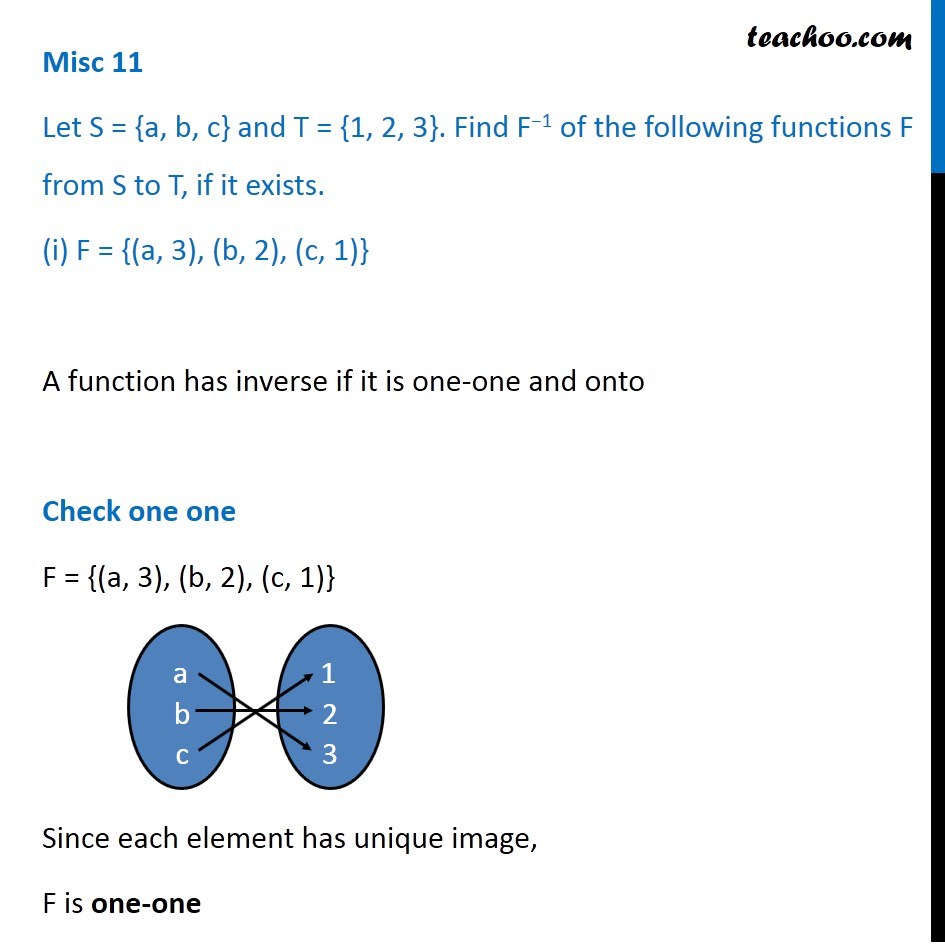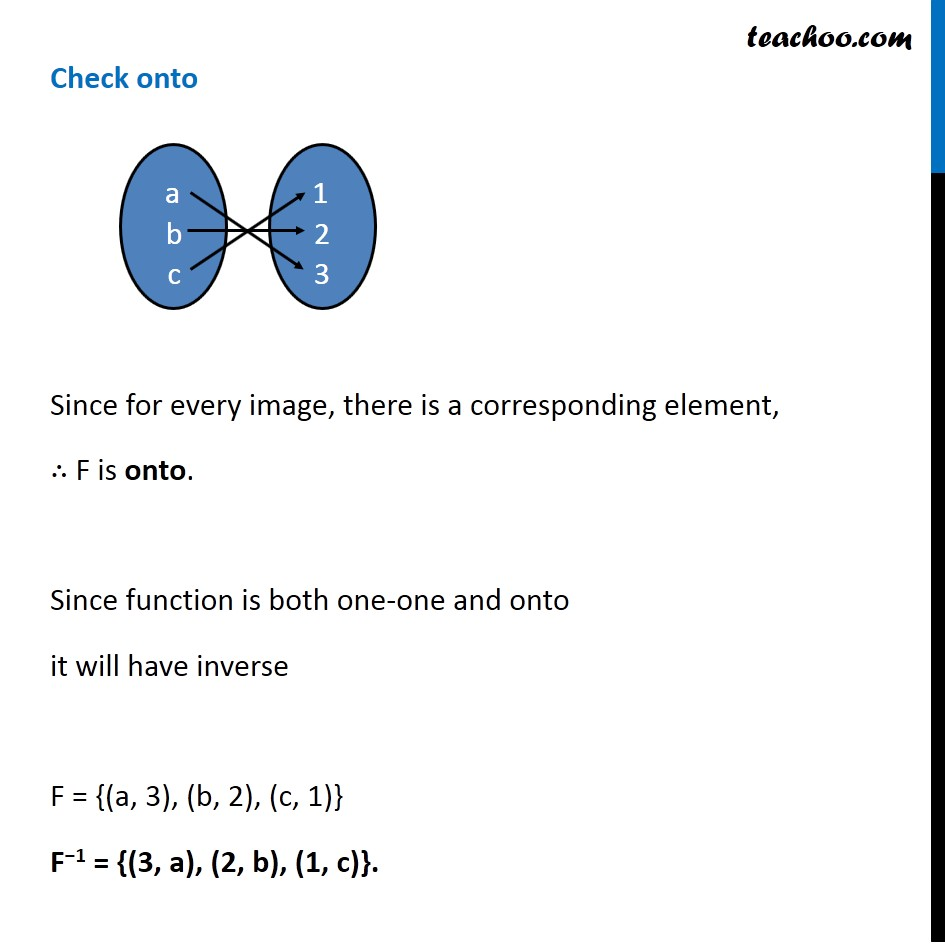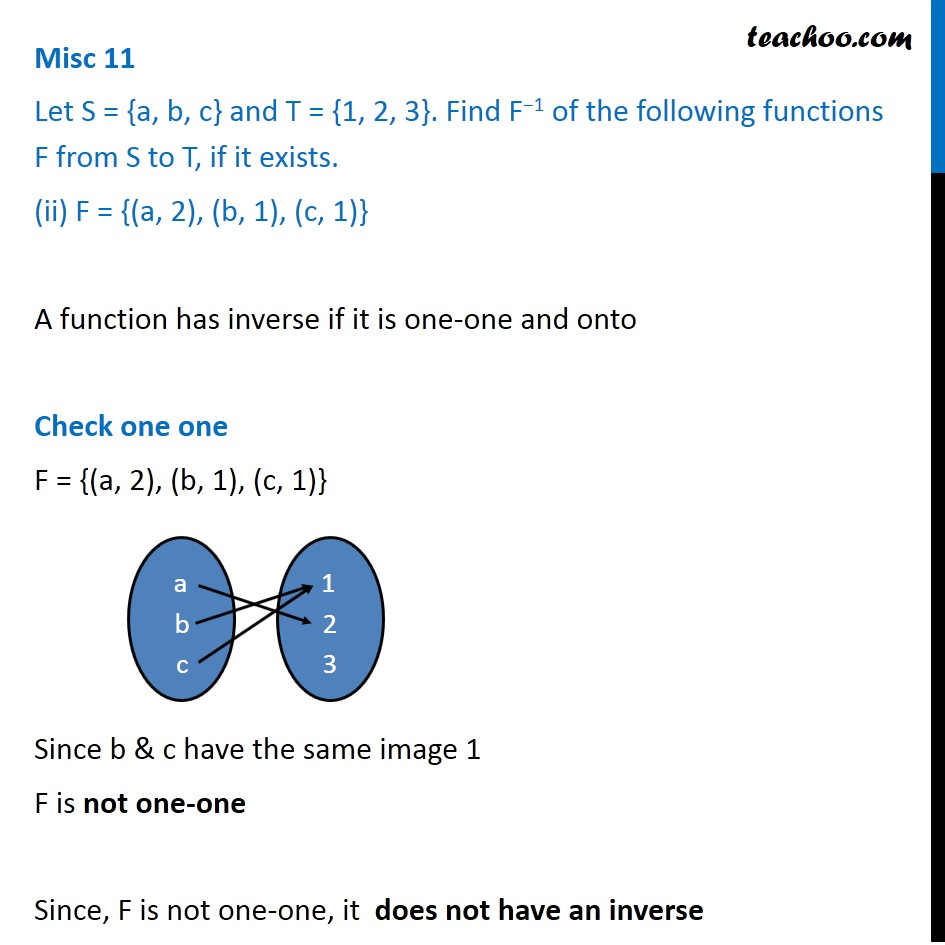Subscribe to our Youtube Channel - https://you.tube/teachoo

1. Chapter 1 Class 12 Relation and Functions
2. Serial order wise
3. Miscellaneous

Transcript

Misc 11 Let S = {a, b, c} and T = {1, 2, 3}. Find F−1 of the following functions F from S to T, if it exists. (i) F = {(a, 3), (b, 2), (c, 1)} A function has inverse if it is one-one and onto Check one one F = {(a, 3), (b, 2), (c, 1)} Since each element has unique image, F is one-one Check onto Since for every image, there is a corresponding element, ∴ F is onto. Since function is both one-one and onto it will have inverse F = {(a, 3), (b, 2), (c, 1)} F−1 = {(3, a), (2, b), (1, c)}. Misc 11 Let S = {a, b, c} and T = {1, 2, 3}. Find F−1 of the following functions F from S to T, if it exists. (ii) F = {(a, 2), (b, 1), (c, 1)} A function has inverse if it is one-one and onto Check one one F = {(a, 2), (b, 1), (c, 1)} Since b & c have the same image 1 F is not one-one Since, F is not one-one, it does not have an inverse

Miscellaneous